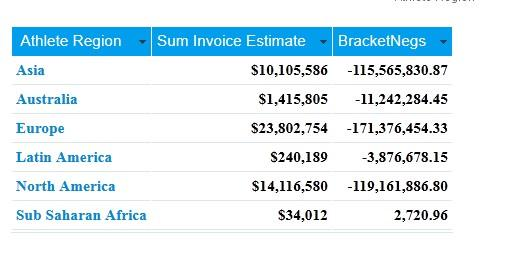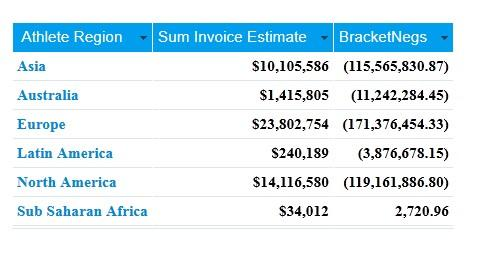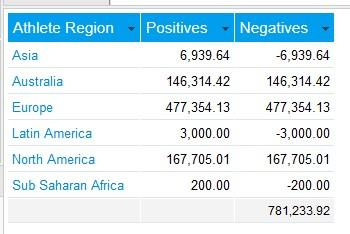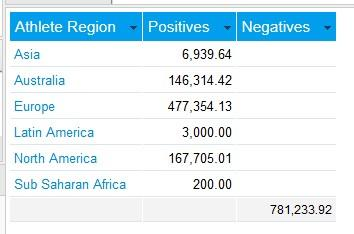# Forum

This content is now out of date.

# How do I display correct Totals when Income and Expenditure figures are both displayed as positive?

Hi

We are preparing financial reports for a client. See attached screenshot.
Client has requested that Operating Expenditure figures show as positive.

I think I can do this using a calculated field based on income/expenditure category.

But is it then possible to establish a Grand Total that is correctly subtracting Expenditure from Income?
Hi Paula,

that sort of requirement is the reason why Yellowfin has the Bracket Negatives option for column formatting:

regular negative values:bracketed negatives:Yellowfin still sees the bracketed numbers as negatives therefore the Grand Total will be correct.

regards,
Dave
Hi David

Yep, we'd started off with the bracketed figures. But the client wants values to show as all positives, with of course totals still calculating correctly.

kind regards

Paula

Hi Paula,

that's a pity, then all I can think of then is:

1) having a main report with the dummied figures (i.e. the non-negative expenditure) with a co-display report directly underneath that shows just the grand total derived from the correct accounting figures.

2) a similar idea as above but done in one report by hiding the correct accounting figures in white font - this works because changing the colour of a column font doesn't affect the grand total, for example

the actual figures:whited out:regards,
Dave
Hi Dave

Thanks for this - this might get us closer to a result.

One more question on this one. Is there a way that I can use an absolute function in a calculated field?

Many thanks

Paula
Hi Paula,

the short answer is yes, if you set the Calculated Field's Formula Type to Freehand SQL then you'll be able to type ABS() or whatever it is for your particular type of database. However, if you're wanting to also use an aggregate function then you won't be able to in a Freehand SQL Calculated Field as they are not allowed.

You could do it if you created a custom-function that contained both the ABS() and SUM() functions, then you could use that by changing the Calculated Field's Formula Type to Pre-Defined.

Or if you just wanted to use the Simple formula type in the Calculated Field (i.e. the Formula Builder) then another idea would be to simulate an ABS() function by using a case statement, something like this:
[code]
CASE WHEN (Sum(income)-Sum(expenditure)) < 0
THEN (Sum(expenditure)-Sum(income))
ELSE (Sum(income)-Sum(expenditure))END[/code]

I hope that's of some help.

regards,
Dave
Thanks very much for your help, Dave.
I'll give it a go.